# Five tractors

Five tractors plow the fields in 4.8 hours. How long will it take to plow the field when, after an hour and a half of work, two tractor drivers have been called up for another task?

x =  7 h

### Step-by-step explanation:Did you find an error or inaccuracy? Feel free to write us. Thank you!Tips to related online calculators
Do you have a linear equation or system of equations and looking for its solution? Or do you have a quadratic equation?
Do you want to convert time units like minutes to seconds?

## Related math problems and questions:

• TractorsFields go plow two tractors with various performances. The first tractor plows the whole field in 11 hours, the second tractor plows the whole field 19 hours longer. How long take plow the whole field with two tractors?
• Tractors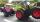Two tractors plow the field in 4 hours together. If the first tractor plow half of the field and then the second tractor completed the job, it would take 9 hours. How many hours does the field plow for each tractor separately?
• Five tractors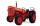Five tractors plow the field in 4.8 hours. How long will it take to plow this field if after 1.5 hours of work, two tractors went to a different field?
• Tractors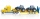5 tractors will plow the field for 8 hours. In 2 hours, 1 tractor broke down. How long do the remaining tractors complete the plow?
• The field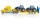3 tractors plow the field in 6 hours. However, after 2 hours, 1 tractor broke down. How long will the field be plowed?
• Tractors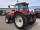Six tractors plowing the field for 8 days. After two days, two tractors broke down. How many days were prolonged work in the field?
• Tractors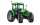Wheel and belt tractors each day plow 8 ha of fields. For plow 76 hectares, the wheel would have to work for 12 days and 8 days with the belt tractor together. How many hectares do each day of each tractor?
• Working time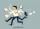At 10 hours work shift, work is done in 16 days. How many days will the work be done at 8 hours shifts?
• Pumps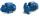Four identical pumps fill the tank in 40 hours. How many pumps would we have to use if we wanted to save 8 hours?
• Painting rooms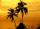If Dano paints three hours daily, he complete in 7.5 days. How many hours a day would have to work to finish the job 1.5 days earlier?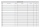Suzan reads a book. If she read half an hour a day, she would read it in nine days. How many minutes does he have to read a day if he wants to read it three days earlier?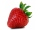Six part-timers would take seven hours to pick strawberries. How long would this work take if two more temporary workers were added after three hours?The work was to be done by 150 workers. At the beginning of their work, their number reduced by 40, which increased the time of work by 5 and 1/3 of the schedule. How long did work take?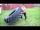Seven tractor plowed 308 ha field in four days. How many hectares of fields will plowe 9 tractors in 3 days at the same power?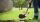Eight diggers will do the job in 85 hours. After 21 hours, 3 diggers had to leave. How many hours will the work be done?The cable car will carry 1280 passengers in 40 minutes. How many people will be transported in 2.5 hours?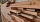Four workers lay on the gym floor in six hours. After an hour of working together, one of the workers went to the doctor. How long will it take for the remaining three workers to complete their work?